# JavaScript: Comparison Operators

## Comparison Operators

Sometimes it is required to compare the value of one variable with other. The comparison operators take simple values (numbers or string) as arguments and evaluate either true or false. Here is a list of comparison operators.

Operator Comparisons
Description
Equal (==) x == y Returns true if the operands are equal.
Strict equal (===) x === y Returns true if the operands are equal and of the same type.
Not equal (!=) x != y Returns true if the operands are not equal.
Strict not equal (!==) x !== y Returns true if the operands are not equal and/or not of the same type.
Greater than (>) x>y Returns true if the left operand is greater than the right operand.
Greater than or equal (>=) x>=y Returns true if the left operand is greater than or equal to the right operand.
Less than (<) x<y Returns true if the left operand is less than the right operand.
Less than or equal (<=) x<=y Returns true if the left operand is less than or equal to the right operand.

## JavaScript Equal (==) operator

Pictorial presentation of Equal (==) operator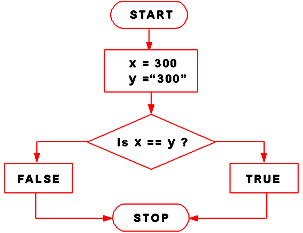```2   ==  2   // true
2   ==  3   // false
2   == '2'  // true
"4" ==  4   // true
```

Example of JavaScript Equal (==) operator

The following function first evaluates if the condition (num == 15) evaluates to true. If it does, it returns the statement between the curly braces (“Equal”). If it doesn’t, it returns the next return statement outside them (“Not equal”).

JavaScript Code:

``````function test_Equal(num) {
if (num == 15) {
return "Equal";
}
return "Not equal";
}
console.log(test_Equal('15')); // "Equal"
console.log(test_Equal(15)); // "Equal"
console.log(test_Equal(25)); // "Not equal"
```
```

## JavaScript Strict Equal (===) operator

Pictorial presentation of Strict equal (===) operator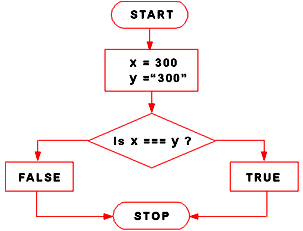```5 ===  5   // true
5 === '5'  // false
```

Example of JavaScript Strict equal (===) operator

The following function first evaluates if the condition (num === 15) evaluates to true. If it does, it returns the statement between the curly braces ("Equal"). If it doesn’t, it returns the next return statement outside them ("Not equal").

JavaScript Code:

``````function test_Strict(num) {
if (num === 15) {
return "Equal";
}
return "Not equal";
}
console.log(test_Strict(15)); // "Equal"
console.log(test_Strict('15')); // "Not equal"
console.log(test_Strict(25)); // "Not equal"
```
```

## JavaScript Not equal (!=) operator

Pictorial presentation of Not equal(!=) operator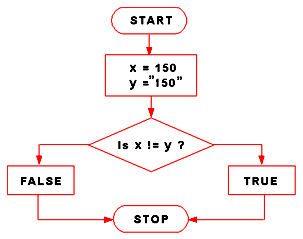```3 !=  4     // true
3 != "3"    // false
3 != '3'    // false
3 != true   // true
0 != false  // false
```

Example of JavaScript Not equal (!=) operator

The following function first evaluates if the condition (num != 55) evaluates to true. If it does, it returns the statement between the curly braces ("Not equal"). If it doesn’t, it returns the next return statement outside them ("Equal").

JavaScript Code:

``````function test_NotEqual(num) {
if (num != 55) {
return "Not Equal";
}
return "Equal";
}
console.log(test_NotEqual(45)); // "Not Equal"
console.log(test_NotEqual(55)); // "Equal"
console.log(test_NotEqual(75)); // "Not Equal"
```
```

## JavaScript Strict not equal (!==) operator

Pictorial presentation of Strict not equal(!==) operator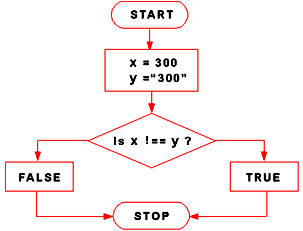```2 !==  2   // false
2 !== '2'  // true
3 !==  2   // true
```

Example of JavaScript Strict Not equal (!==) operator

The following function first evaluates if the condition (num !== 15) evaluates to true considering both value and value type. If it does, it returns the statement between the curly braces ("Not equal"). If it doesn’t, it returns the next return statement outside them ("Equal").

JavaScript Code:

``````function test_StrictNotEqual(num) {
if (num !== 15) {
return "Not equal";
}
return "Equal";
}
console.log(test_StrictNotEqual(15)); // "Equal"
console.log(test_StrictNotEqual(20)); // "Not equal"
console.log(test_StrictNotEqual(25)); // "Not equal"
```
```

## JavaScript Greater than(>) operator

Pictorial presentation of Greater than(>) operator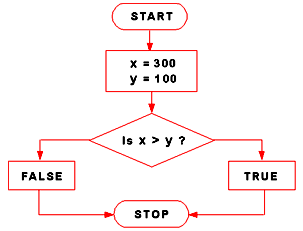```6   >  4   // true
8   > '4'  // true
3   >  2   // true
'2' >  5   // false
```

Example of JavaScript Greater than(>) operator

The following function first evaluates if the condition (num > 50) evaluates to true converting num to a number if necessary. If it does, it returns the statement between the curly braces ("Over 50"). If it doesn’t, it checks if the next condition is true (returning "Over 15"). Otherwise the function will return "10 or under".

JavaScript Code:

``````function test_GreaterThan(num) {
if (num > 50) {
return "Over 50";
}
if (num > 15) {
return "Over 15";
}
return "10 or under";
}
console.log(test_GreaterThan(70));  // "Over 50"
console.log(test_GreaterThan(10)); //  "10 or under"
console.log(test_GreaterThan(45)); // "Over 15"
```
```

## JavaScript Greater than or equal (>=) operator

Pictorial presentation of Greater than or equal (>=) operator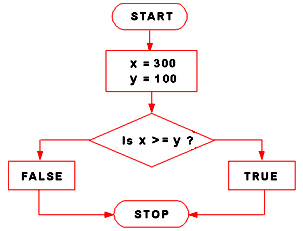```5   >=  5   // true
8   >= '4'  // true
3   >=  5   // false
'5' >=  7   // false
```

Example of JavaScript Greater than or equal (>=) operator

The following function first evaluates if the condition (num >= 50) evaluates to true converting num to a number if necessary. If it does, it returns the statement between the curly braces ("50 or Over"). If it doesn’t, it checks if the next condition is true (returning "20 or Over"). Otherwise the function will return "Less than 20".

JavaScript Code:

``````function test_GreaterOrEqual(num) {
if (num >= 50) return "50 or Over";

if (num >= 20) return "20 or Over";

return "Less than 20";
}
console.log(test_GreaterOrEqual(70)); // "50 or Over"
console.log(test_GreaterOrEqual(10)); // "Less than 20"
console.log(test_GreaterOrEqual(45)); // "20 or Over"
``````

## JavaScript Less than (<) operator

Pictorial presentation of Less than (<) operator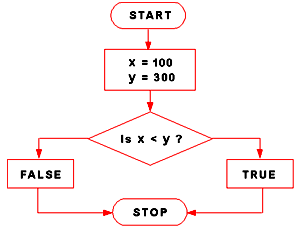```3   < 6  // true
'3' < 5  // true
6   < 6  // false
4   < 3  // false
'5' < 3  // false
```

The following function first evaluates if the condition (num < 50) evaluates to true converting num to a number if necessary. If it does, it returns the statement between the curly braces ("Less than 50"). If it doesn’t, it checks if the next condition is true (returning "Less than 15"). Otherwise the function will return "10 or under".

JavaScript Code:

``````function test_GreaterThan(num) {
if (num < 50) {
return "Less than 50";
}
if (num < 15) {
return "Less than 15";
}
return "10 or under";
}
console.log(test_GreaterThan(70));  // "Less than 50"
console.log(test_GreaterThan(10)); //  "Less than 15"
console.log(test_GreaterThan(45)); // "10 or under"``````

## JavaScript Less than or equal (<=) operator

Pictorial presentation of Less than or equal (<=) operator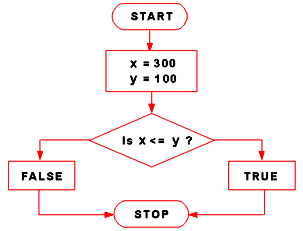```5   <= 6  // true
'8' <= 8  // true
6   <= 6  // true
5   <= 3  // false
'6' <= 2  // false
```

Example of JavaScript Less than or equal (<=) operator

The following function first evaluates if the condition (num <=25) evaluates to true converting num to a number if necessary. If it does, it returns the statement between the curly braces ("Smaller Than or Equal to 25"). If it doesn’t, it checks if the next condition is true (returning "Smaller Than or Equal to 25"). Otherwise the function will return "More Than 50".

JavaScript Code:

``````function test_LessOrEqual(num) {
if (num <= 25) return "Smaller Than or Equal to 25";

if (num <= 50) return "Smaller Than or Equal to 50";

return "More Than 50";
}
console.log(test_LessOrEqual(50));  // "Smaller Than or Equal to 50"
console.log(test_LessOrEqual(10));  // "Smaller Than or Equal to 25"
console.log(test_LessOrEqual(75));  // "More Than 50"
```
```

JavaScript Code Editor:

See the Pen JavaScript-blank-editor-operator by w3resource (@w3resource) on CodePen.

Test your Programming skills with w3resource's quiz.

﻿

## JavaScript: Tips of the Day

Memoized function

```const add = () => {
const cache = {};
return num => {
if (num in cache) {
return 'From cache! \${cache[num]}';
} else {
const result = num + 10;
cache[num] = result;
return 'Calculated! \${result}';
}
};
};